热图怎么做？ | 热图函数pheatmap()

pheatmap(mat, color = colorRampPalette(rev(brewer.pal(n = 7, name =

“RdYlBu”)))(100), kmeans_k = NA, breaks = NA, border_color = “grey60”,

cellwidth = NA, cellheight = NA, scale = “none”, cluster_rows = TRUE,

cluster_cols = TRUE, clustering_distance_rows = “euclidean”,

clustering_distance_cols = “euclidean”, clustering_method = “complete”,

clustering_callback = identity2, cutree_rows = NA, cutree_cols = NA,

treeheight_row = ifelse((class(cluster_rows) == “hclust”) || cluster_rows,

50, 0), treeheight_col = ifelse((class(cluster_cols) == “hclust”) ||

cluster_cols, 50, 0), legend = TRUE, legend_breaks = NA,

legend_labels = NA, annotation_row = NA, annotation_col = NA,

annotation = NA, annotation_colors = NA, annotation_legend = TRUE,

annotation_names_row = TRUE, annotation_names_col = TRUE,

drop_levels = TRUE, show_rownames = T, show_colnames = T, main = NA,

fontsize = 10, fontsize_row = fontsize, fontsize_col = fontsize,

display_numbers = F, number_format = “%.2f”, number_color = “grey30”,

fontsize_number = 0.8 * fontsize, gaps_row = NULL, gaps_col = NULL,

labels_row = NULL, labels_col = NULL, filename = NA, width = NA,

height = NA, silent = FALSE, na_col = “#DDDDDD”, …)

第1步：数据准备

• mat：用来画热图的数据参数，一般是一个矩阵，数据是基因表达值，行代表基因，列代表样本。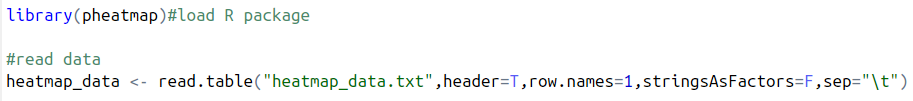第2步：画图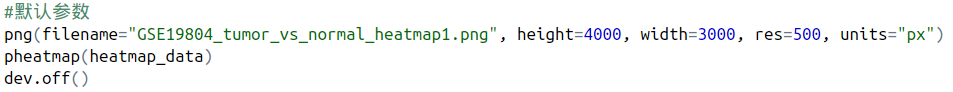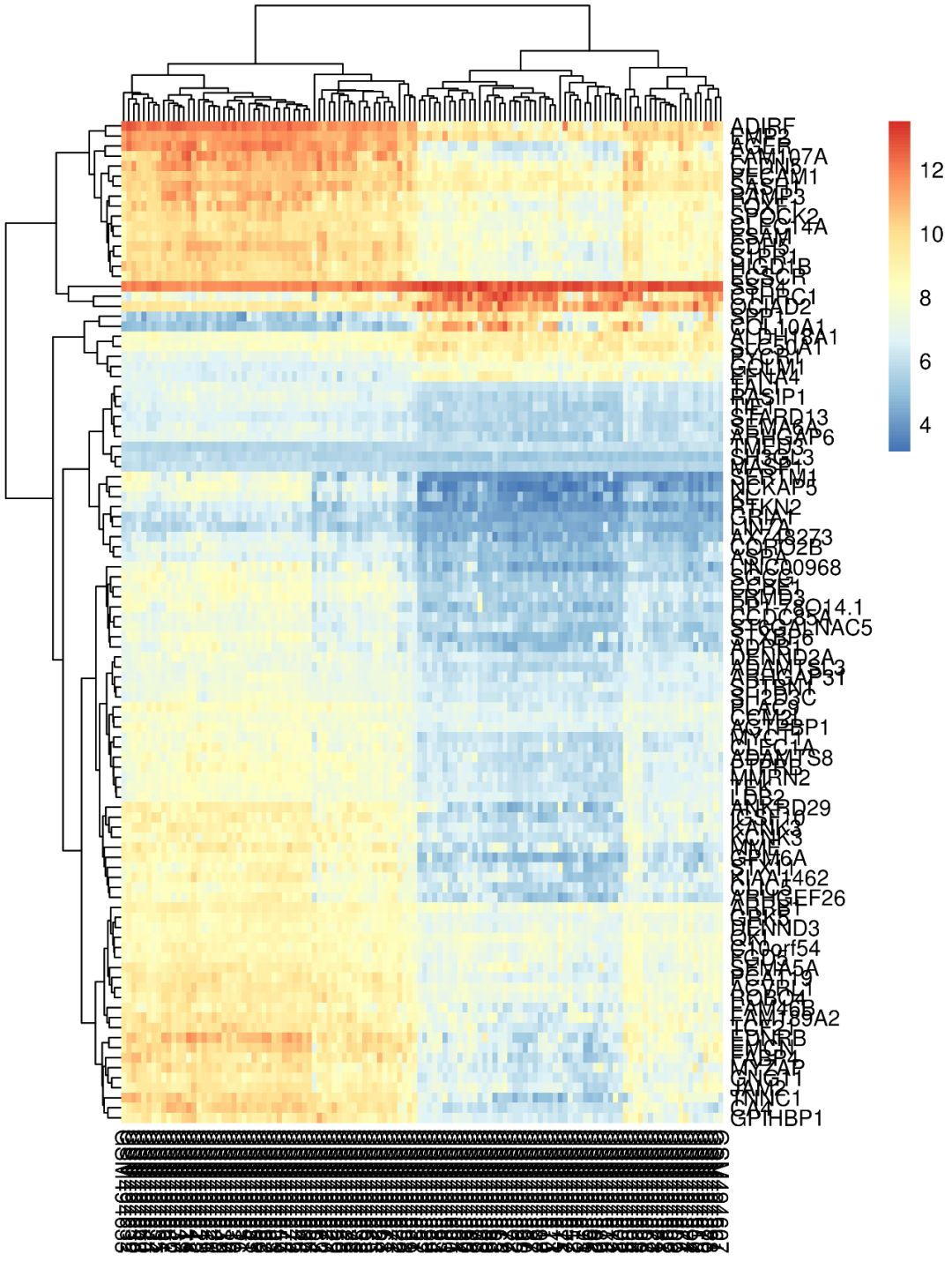第3步：参数调整

• color：表示颜色，用来画热图的颜色，可以自己定义，默认值为colorRampPalette(rev(brewer.pal(n = 7, name =”RdYlBu”)))(100)，RdYlBu也就是Rd红色，Yi黄色，Bu蓝色的过度，则主调色为红黄蓝。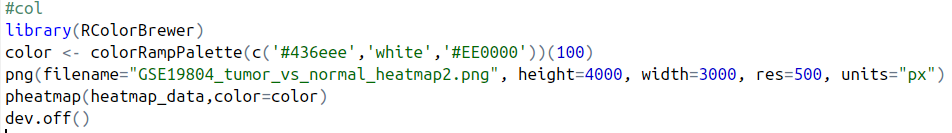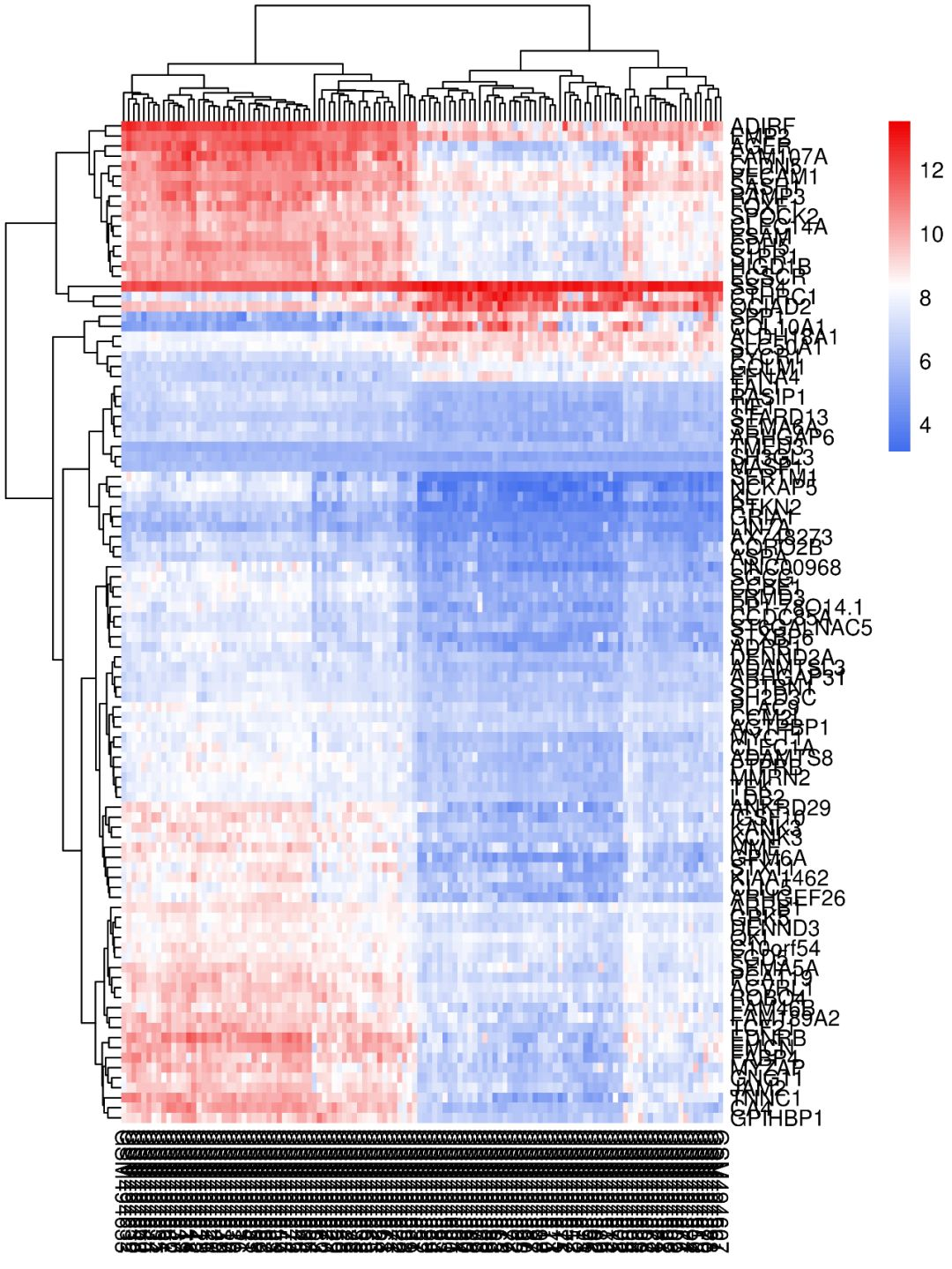• scale：是指对数值进行均一化处理，在基因表达量的数据中，有些基因表达量极低，有些基因表达量极高，因此把每个基因在不同处理和重复中的数据转换为平均值为0，方差为1的数据，可以看出每个基因在某个处理和重复中表达量是高还是低，一般选择做row均一化。
• clustering_method：表示聚类方法，值可以是hclust的任何一种，如”ward.D”,”single”, “complete”, “average”, “mcquitty”, “median”, “centroid”, “ward.D2″。
• cluster_rows：表示行是否聚类，值可以是FALSE或TRUE
• clustering_distance_rows：行距离度量的方法，如欧氏距离
• cutree_rows：行聚类数
• treeheight_row：行聚类树的高度，默认为50
• gaps_row：对行进行分割，就不应对相应的行进行聚类
• cluster_cols：表示列是否聚类，值可以是FALSE或TRUE
• clustering_distance_cols：列距离度量的方法
• cutree_cols：列聚类数
• treeheight_col：列聚类树的高度，默认为50
• gaps_col：对列进行分割，就不应对相应的列进行聚类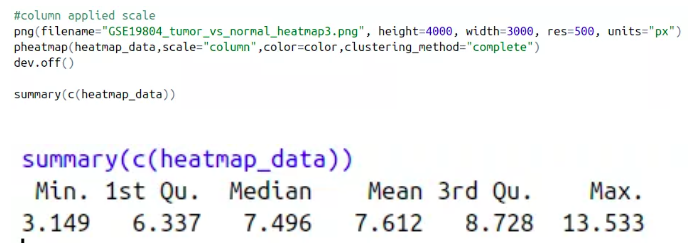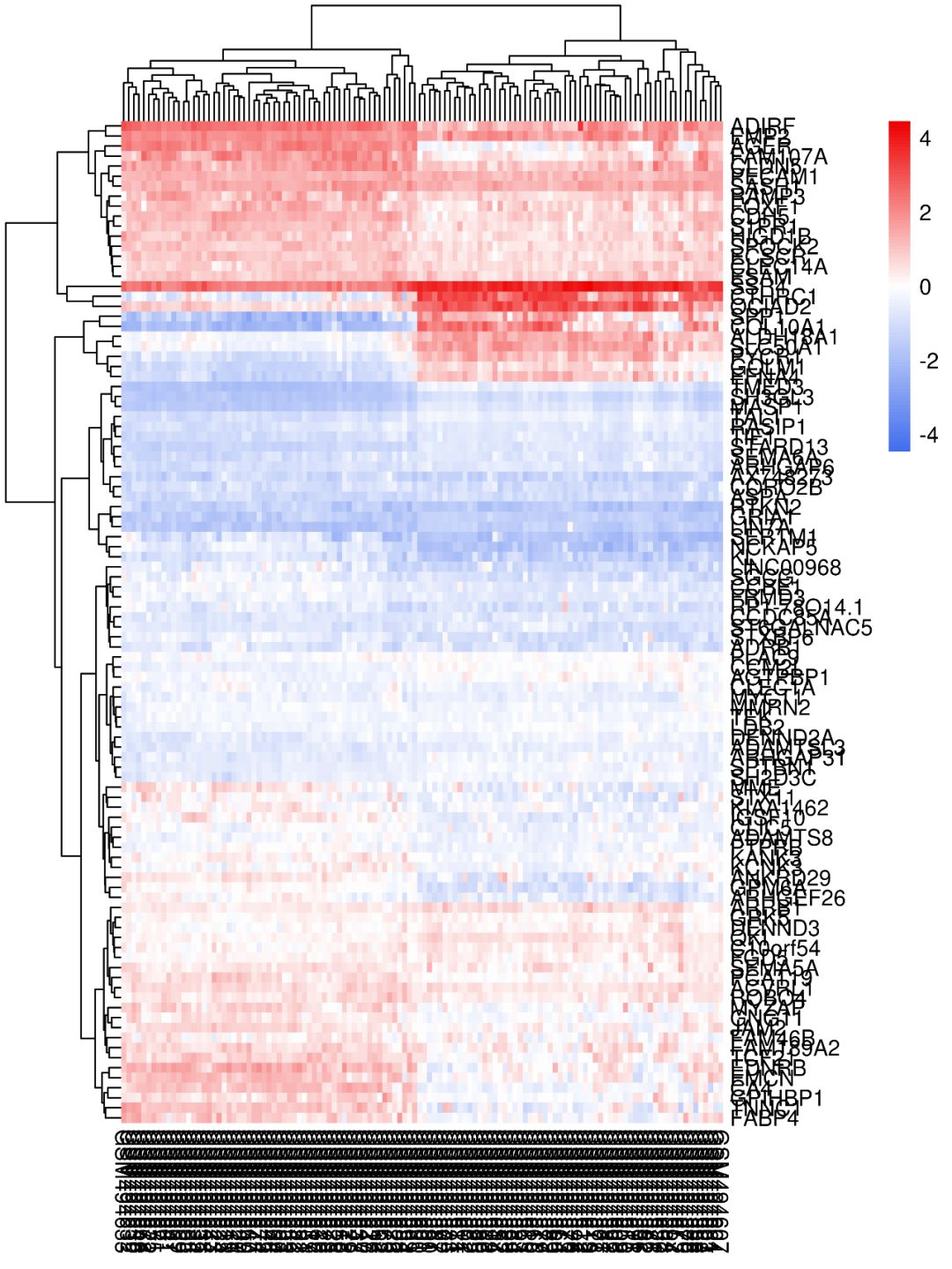• legend：逻辑值，是否显示色度条，默认为T
• legend_breaks：显示多少个颜色数值段
• legend_labels：对色度条上对应位置的字符进行修改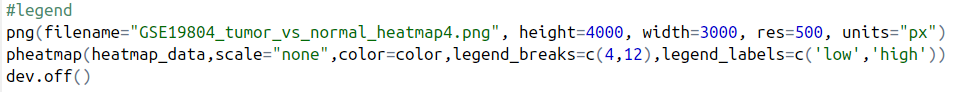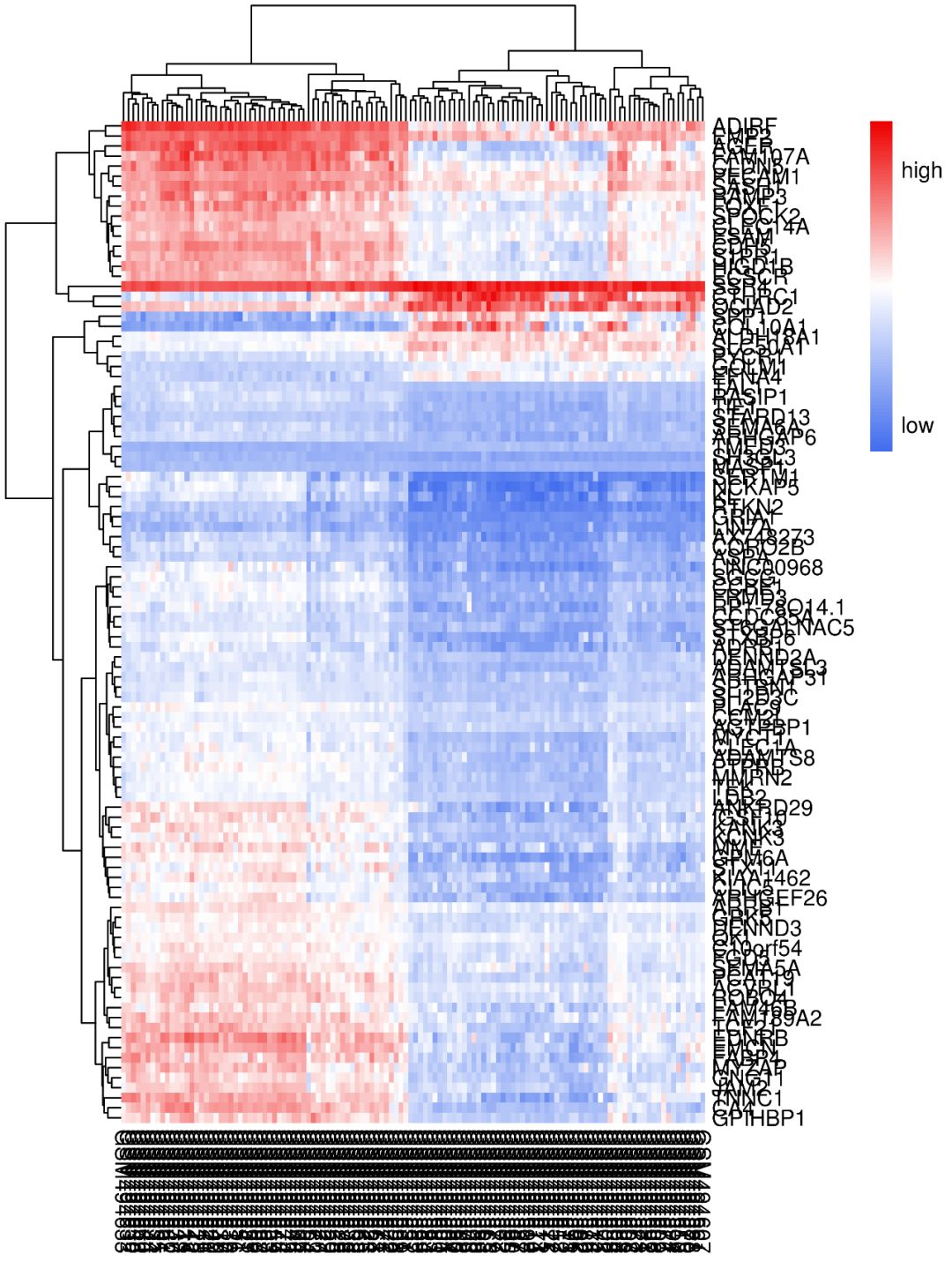• annotation_colors：对标签的颜色进行修改
• annotation_legend：是否显示标签注释条
• annotation_row：数据框格式，用来定义热图所在行的注释条
• annotation_names_row：逻辑值，是否显示行标签名称
• annotation_col：数据框格式，用来定义热图所在列的注释条
• annotation_names_col：逻辑值，是否显示列标签名称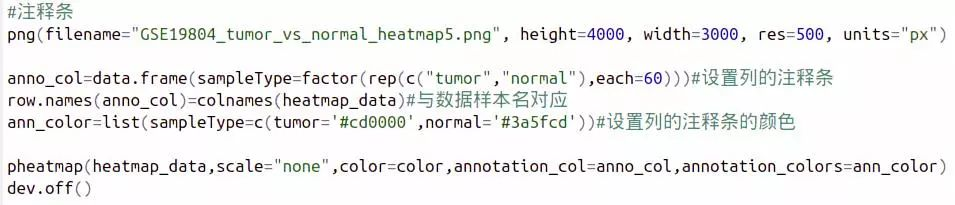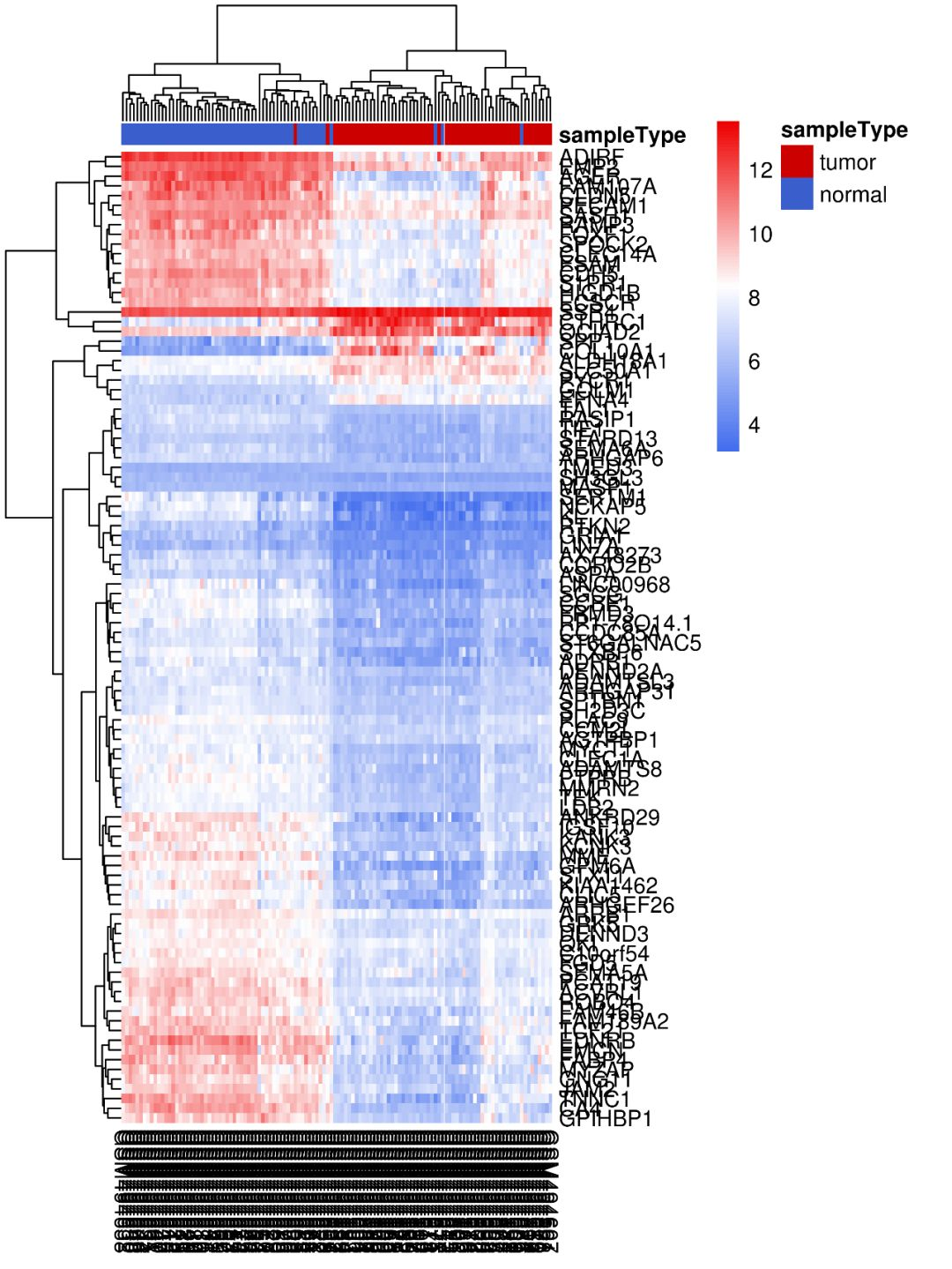• main：设置图的标题
• fontsize：是设置所有除主图以外的标签的大小
• number_color：字体的颜色
• show_rownames：是否显示行名
• fontsize_row：行名的字体大小
• labels_row：X轴坐标名设置
• show_colnames：是否显示列名
• fontsize_col：列名的字体大小
• labels_col：y轴坐标名设置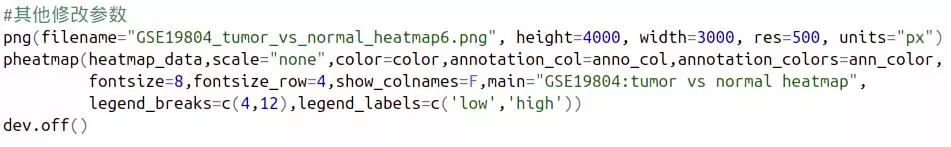• fontsize_number：小格子中数字大小
• display_numbers：逻辑值，是否在小格子中显示数字
• number_format：小格子中数字显示形式，但仅有在display_numbers=T时才能使用
• na_col：设置小格子为缺失值时的颜色
• cellwidth：表示每个小格子的宽度
• cellheight：表示每个小格子的高度

• filename：输出图画的文件名
• width：输出图画的宽度
• height：输出图画的高度